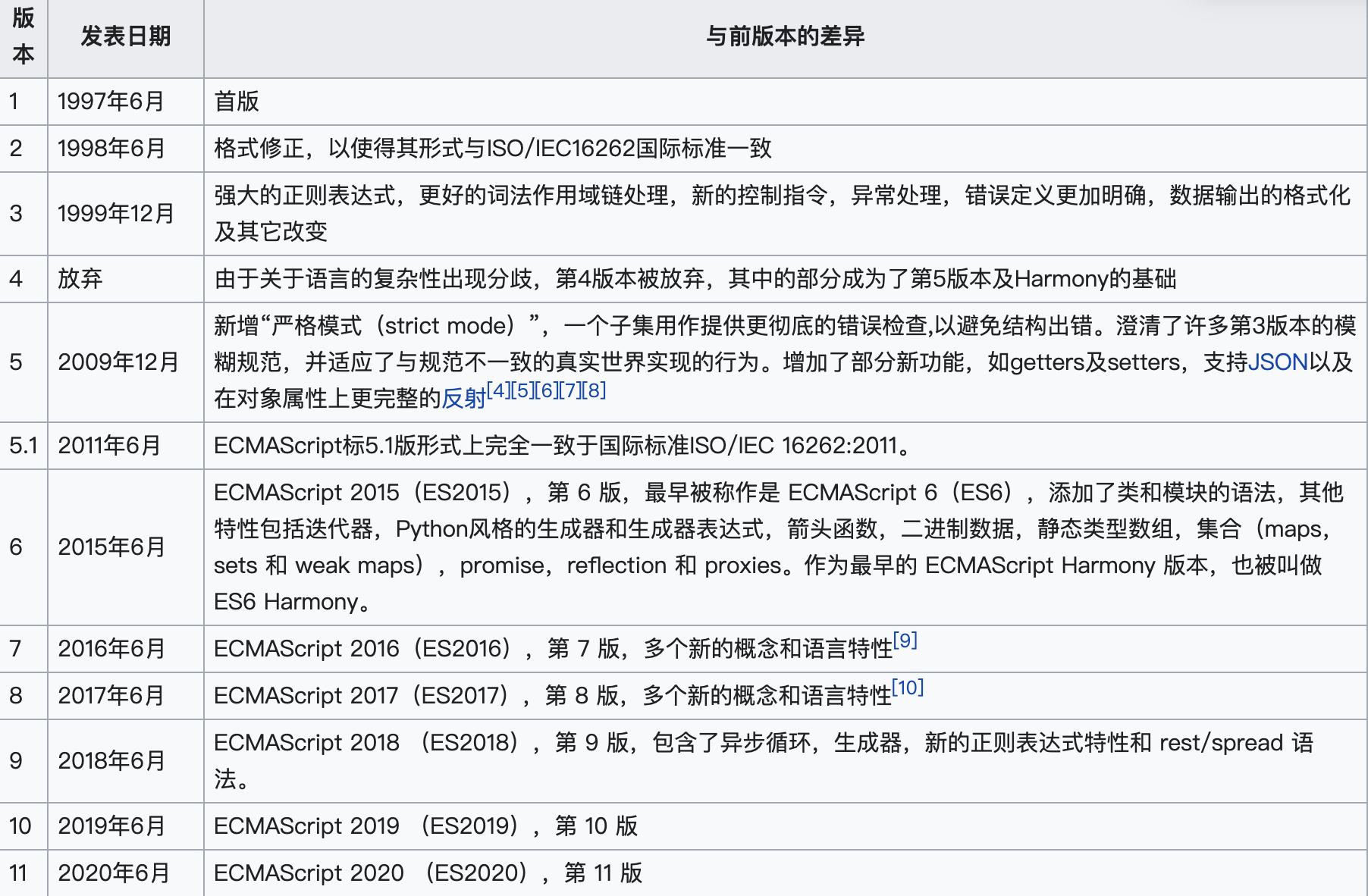# 简介

ECMAScript 6.0（以下简称 ES6）是 JavaScript 语言的下一代标准，正式发布与2015年6月。它的目标，是使得JavaScript语言可以用来编写复杂的大型应用程序，成为企业级开发语言。

# ECMAScript和JavaScript的关系

1996年11月，JavaScript 的创造者 Netscape 公司，决定将 JavaScript 提交给国际标准化组织ECMA.

1997年, ECMA 发布262号标准文件 ECMAScript 1.0。

ECMAScript 和 JavaScript 的关系是，前者是后者的规格，后者是前者的一种实现。# let和const

ES6中引入了let和const,是为了解决之前的var变量的种种问题。

1. 变量提升

var命令会发生”变量提升”现象，即变量可以在声明之前使用，值为undefined.

``````// var 的情况
console.log(foo);  // 输出undefined
var foo = 2;
``````
1. 变量覆盖

``````var tmp = new Date();
function f() {
console.log(tmp);
if (false) { var tmp = "hello world";
} }
f(); // undefined
``````
1. 变量泄露

``````var s = 'hello';
for (var i = 0; i < s.length; i++) {
console.log(s[i]);
}
console.log(i); // 5
~~

~~~js
{
let a = 10;
var b = 1;
}
a // ReferenceError: a is not defined.
b // 1
``````

# 解构赋值

ES6 允许按照一定模式，从数组和对象中提取值，对变量进行赋值，这被称为解构。

``````let [a, b, c] = [1, 2, 3];
let [ , , third] = ["foo", "bar", "baz"];
let [x, , y] = [1, 2, 3];
let [head, ...tail] = [1, 2, 3, 4];

let [x, y] = [1, 2, 3];

``````

``````let [foo = true] = [];
foo // true

let [x, y = 'b'] = ['a'];
// x='a', y='b'

let [x, y = 'b'] = ['a', undefined];
// x='a', y='b’

let [x = 1] = [undefined];
x // 1
let [x = 1] = [null];
x // null
``````

``````function f() {
console.log('aaa');
}

let [x = f()] = ;
``````

``````let { bar, foo } = { foo: "aaa", bar: "bbb" };
foo // "aaa"
bar // "bbb"

let { baz } = { foo: "aaa", bar: "bbb" };
baz // undefined

var { foo: baz } = { foo: 'aaa', bar: 'bbb' };
baz // "aaa"

let obj = { first: 'hello', last: 'world' };
let { first: f, last: l } = obj;
f // 'hello'
l // 'world'
``````

``````let obj = { p: [ 'Hello', { y: 'World' } ] };

let { p: [x, { y }] } = obj;

x // "Hello"
y // "World"
``````

``````let x = 1;
let y = 2;
[x, y] = [y, x];
``````

``````// 返回一个数组
function example() { return [1, 2, 3]; }
let [a, b, c] = example();

// 返回一个对象
function example() { return { foo: 1, bar: 2 }; }
let { foo, bar } = example();

//提取JSON数据
let jsonData = { id: 42, status: "OK", data: [867, 5309] };
let { id, status, data: number } = jsonData;
``````

# 数组的扩展

ES6中的Array.from方法用于将下面两类对象转为真正的数组：

• 类似数组的对象（array-like object）
• 可遍历（iterable）的对象（包括ES6新增的数据结构Set和Map）。

``````let arrayLike = { '0': 'a', '1': 'b', '2': 'c', length: 3 };
``````

``````// ES5的写法
var arr1 = [].slice.call(arrayLike);
// ['a', 'b', 'c']

// ES6的写法 let arr2 = Array.from(arrayLike);
// ['a', 'b', 'c']
``````

``````// NodeList对象
let ps = document.querySelectorAll('p');
Array.from(ps).forEach(function (p) { console.log(p); });

// arguments对象
function foo() { var args = Array.from(arguments);
// ...
}
``````

``````Array.from('hello') // ['h', 'e', 'l', 'l', 'o']
let namesSet = new Set(['a', 'b'])
Array.from(namesSet) // ['a', 'b']
``````

``````function foo() { var args = [...arguments]; } // arguments对象
[...document.querySelectorAll('div')] // NodeList对象
``````

Array.from方法还可以接收第二个参数，用来对数组中的元素进行操作：

``````Array.from(arrayLike, x => x * x);
// 等同于
Array.from(arrayLike).map(x => x * x);

Array.from([1, 2, 3], (x) => x * x)
// [1, 4, 9]
``````

Array.of方法可以很方便的创建新的数组：

``````Array.of(3, 11, 8) // [3,11,8]
Array.of(3) // 
Array.of(3).length // 1

Array() // []
Array(3) // [, , ,]
Array(3, 11, 8) // [3, 11, 8]
``````

# 函数的扩展

ES6，可以支持函数的默认值了：

``````function log(x, y = 'World') { console.log(x, y); }
function Point(x = 0, y = 0) { this.x = x; this.y = y; }
``````

``````function foo({x, y = 5}) { console.log(x, y); }
foo({}) // undefined, 5
foo({x: 1}) // 1, 5
foo({x: 1, y: 2}) // 1, 2
foo() // TypeError: Cannot read property 'x' of undefined
``````

``````// 写法一
function m1({x = 0, y = 0} = {})
{ return [x, y]; }

// 写法二
function m2({x, y} = { x: 0, y: 0 })
{ return [x, y]; }
``````

``````m1() // [0, 0]
m2() // [0, 0]
``````

``````m1({x: 3, y: 8}) // [3, 8]
m2({x: 3, y: 8}) // [3, 8]
``````

``````m1({x: 3}) // [3, 0]
m2({x: 3}) // [3, undefined]
``````

``````m1({}) // [0, 0];
m2({}) // [undefined, undefined]
m1({z: 3}) // [0, 0]
m2({z: 3}) // [undefined, undefined]
``````